Mathematics

# Onto Function

5.5k views

Table of Contents

 1 Introduction 2 Onto Function 3 What does it mean for a function to be onto? 4 Number of Onto Functions 5 Prove a function is onto 6 Surjective Function Examples 7 Summary 8 FAQs

22 December 2020

Read time: 8 minutes

## Introduction

Do you know – what is a function?

By the word function, we may understand the responsibility of the role one has to play. For example, the function of the leaves of plants is to prepare food for the plant and store them. Similarly, the function of the roots of the plants is to absorb water and other nutrients from the ground and supply it to the plants and help them stand erect.

Can we say that everyone has different types of functions?

Let’s try to learn the concept behind one of the types of functions in mathematics!
And particularly onto functions.

### Onto functions-PDF

Cuemath’s learning platform has carefully designed simulations to help the student visualize concepts. This blog will help us understand about the Onto functions. Here is a downloadable PDF to explore more.

 📥 Onto functions-PDF Download

Also read:

In mathematics, a function means a correspondence from one value x of the first set to another value y of the second set. This correspondence can be of the following four types. But each correspondence is not a function.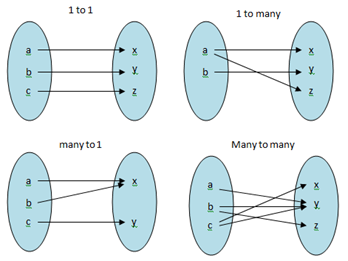In the above figure, only 1 – 1 and many to one are examples of a function because no two ordered pairs have the same first component and all elements of the first set are linked in them.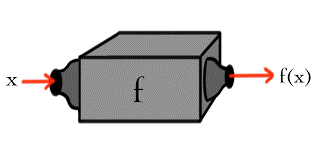So we say that in a function one input can result in only one output. If we are given any x then there is one and only one y that can be paired with that x.
The following diagram depicts a function: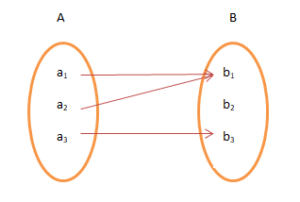A function is a specific type of relation. Any relation may have more than one output for any given input. For example:-

• The temperature on any day in a particular City.

• The number of calories intakes by the fast food you eat.

• A number of places you can drive to with only one gallon left in your petrol tank.

• The number of sodas coming out of a vending machine depending on how much money you insert.

• The amount of carbon left in a fossil after a certain number of years.

• The height of a person at a specific age.

But for a function, every x in the first set should be linked to a unique y in the second set. So examples 1, 2, and 3 above are not functions. And examples 4, 5, and 6 are functions.

### Types of functions

If a function does not map two different elements in the domain to the same element in the range, it is called a one-to-one or injective function.

If a function has its codomain equal to its range, then the function is called onto or surjective. In this article, we will learn more about functions.

## Onto Function

A function f: A $$\rightarrow$$ B is termed an onto function if

The range that exists for f is the set B itself.

### What is an onto function?

In other words, if each y ∈ B there exists at least one x ∈ A such that

f(y) = x,

then f is an onto function. An onto function is also called a surjective function.

Let A = {a1 , a2 , a3 } and B = {b1 , b2 } then f : A →B.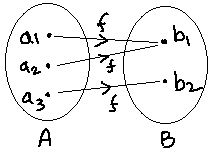## What does it mean for a function to be onto

ONTO-ness is a very important concept while determining the inverse of a function.

 BOTH 1-1 & Onto Functions A function f from A (the domain) to B (the codomain) is BOTH one-to-one and onto when no element of B is the image of more than one element in A, AND all elements in B are used as images. Such functions are called bijective and are invertible functions.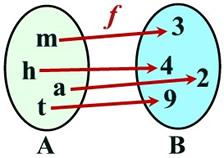Function f: BOTH One-to-one and Onto Each used element of B is used only once, and All elements in B are used.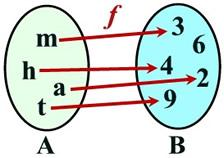Function f: NOT BOTH it is One-to-one but NOT onto Each used element of B is used only once, but the 6 in B is not used.

So the first one is invertible and the second function is not invertible.

## Number of Onto Functions

Number of onto functions from a to b?

If Set A has m elements and Set B has  n elements then  Number  of surjections (onto function) are

• ncm* m !, if n ≥ m
• 0, if  n < m
 Example

How many onto functions are possible from a set containing m elements to another set containing 2 elements?

Solution

From a set having m elements to a set having 2 elements, the total number of functions possible is 2m. Out of these functions, 2 functions are not onto (viz. If all elements are mapped to the 1st element of Y or if all elements are mapped to the 2nd element of Y). So, subtracting it from the total number of functions we get, the number of onto functions as 2m-2.

### The number of onto functions

For finite sets A and B $$|A|=M$$ and $$|B|=n,$$ the number of onto functions is: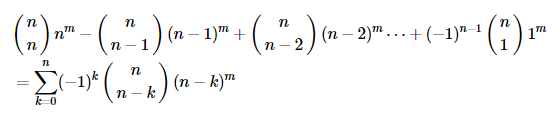Example

The number of surjective functions from set X = {1, 2, 3, 4} to set Y = {a, b, c} is:
(A) 36
(B) 64
(C) 81
(D) 72

Solution

Using m = 4 and n = 3, the number of onto functions is:

 34 – 3C1(2)4 + 3C214 = 36.

## Prove a Function is Onto

For proving a function to be onto we can either prove that range is equal to codomain or just prove that every element y ε codomain has at least one pre-image x ε domain.

### How to tell if a function is onto?

 Example 1

Let A = {1, 2, 3}, B = {4, 5} and let f = {(1, 4), (2, 5), (3, 5)}. Check if f is a surjective function from A into B.

Solution

Domain = A = {1, 2, 3}

we see that the element from A,

1 has an image 4, and both 2 and 3 have the same image 5.

Thus the Range of the function is {4, 5} which is equal to B.

So we conclude that f : A →B  is an onto function.

 Example 2

How to tell if a function is onto? f : R → R  defined by f(x)=1+x2.

Solution

f(x)=1+x2.

for real number we know that x2 >0

So 1+x2 >1

f(x) > 1 and hence the range of the function is (1, ∞)

Whereas, the second set is R (Real Numbers). So range is not equal to codomain and hence the function is not onto.

 Example 3

Is f(x)=3x−4 an onto function where $$f: \mathbb{R}\rightarrow \mathbb{R}$$?

Solution: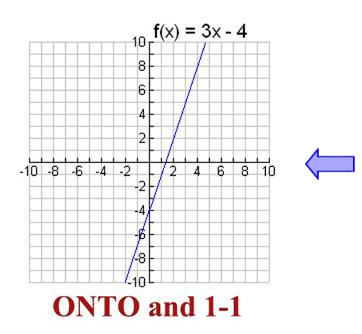This function (which is a straight line) is ONTO.

We see that as we progress along the line, every possible y-value from the codomain has a pre-linkage.

In addition, this straight line also possesses the property that each x-value has one unique y- value that is not used by any other x-element. This function is also one-to-one.

## Surjective Function Examples

To see some of the surjective function examples, let us keep trying to prove a function is onto.

Let us look into a few more examples and how to prove a function is onto.

 Example 4

Prove a function is onto.

Is g(x)=x2−2 an onto function where $$g: \mathbb{R}\rightarrow \mathbb{R}$$?

Solution: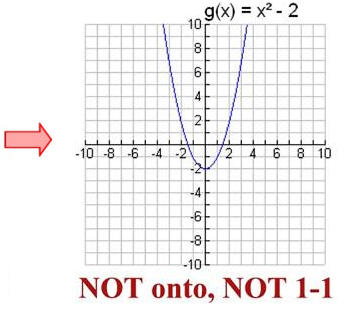The graph of this function (results in a parabola) is NOT ONTO.

From the graph, we see that values less than -2 on the y-axis are never used. Since only certain y-values (i.e. [2, ∞)) are used, we see that not all possible y-values have a pre-image.

Thus, this is not an onto function.

 Example 5

Prove a function is onto. Is g(x)=x2−2  an onto function where $$g: \mathbb{R}\rightarrow [-2, \infty)$$ ?

Solution:

If set B, the codomain, is redefined to be , from the above graph we can say, that all the possible y-values are now used or have at least one pre-image, and function g (x) under these conditions is ONTO.

### Check your understanding

Question 1: Determine which of the following functions f: R →R  is an onto function

• f(x)=x=1
• f(x)=|x|+x

## Summary

A function is onto when its range and codomain are equal. We can also say that function is onto when every y ε codomain has at least one pre-image x ε domain.

In this blog we have discussed about:

• Onto Function

• What does it mean for a function to be onto?

• Number of Onto Functions

• Prove a function is onto

• Surjective Function Examples

We believe in better leaning…... So I hope you have understood about onto functions in detail from this article.

Written by Anupama Mahajan

## About Cuemath

Cuemath, a student-friendly mathematics and coding platform, conducts regular Online Live Classes for academics and skill-development, and their Mental Math App, on both iOS and Android, is a one-stop solution for kids to develop multiple skills. Understand the Cuemath Fee structure and sign up for a free trial.

## What is a surjective function?

A function f : A → B  is termed an onto function if

The range that exists for f is the set B itself.

In other words, if each y ∈ B there exists at least one x ∈ A  such that

f(y)=x,

then f is an onto function. An onto function is also called a surjective function.
Let A = {a1 , a2 , a3 } and B = {b1 , b2 } then f : A → B.Codomain = Range

## How do you prove that a function is onto?

By proving Range equal to codomain

No

## External References

To know more about Onto functions, visit these blogs:

GIVE YOUR CHILD THE CUEMATH EDGE
Access Personalised Math learning through interactive worksheets, gamified concepts and grade-wise courses
Learn More About Cuemath